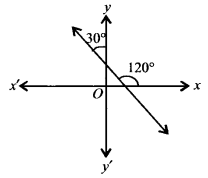NCERT Solutions for Class 11 Maths Chapter 10 Straight Lines Ex 10.1

# NCERT Solutions for Class 11 Maths Chapter 10 Straight Lines Ex 10.1

## NCERT Solutions for Class 11 Maths Chapter 10 Straight Lines Ex 10.1

NCERT Solutions for Class 11 Maths Chapter 10 Straight Lines Ex 10.1 are the part of NCERT Solutions for Class 11 Maths. Here you can find the NCERT Solutions for Class 11 Maths Chapter 10 Straight Lines Ex 10.1.

### Ex 10.1 Class 11 Maths Question 1.

Draw a quadrilateral in the Cartesian plane, whose vertices are (-4, 5), (0, 7), (5, -5) and (-4, -2). Also, find its area.

Solution:
The figure of quadrilateral whose vertices are A(-4, 5), B(0, 7), C(5, -5) and D(-4, -2) is given below.

### Ex 10.1 Class 11 Maths Question 2.

The base of an equilateral triangle with side 2a lies along the y-axis such that the mid-point of the base is at the origin. Find vertices of the triangle.

Solution:
Since base of an equilateral triangle lies along y-axis.

### Ex 10.1 Class 11 Maths Question 3.

Find the distance between P(x1, y1) and Q(x2, y2) when:
(i) PQ is parallel to the y-axis,
(ii) PQ is parallel to the x-axis.

Solution:
It is given that the coordinates of P is (x1, y1) and the coordinates of Q is (x2, y1).
The distance between the points P(x1, y1) and Q(x2, y2) is:### Ex 10.1 Class 11 Maths Question 4.

Find a point on the x-axis, which is equidistant from the points (7, 6) and (3, 4).

Solution:
Let the point be P(x, y).

Since, the point lies on the x-axis.

y = 0, i.e., the required point is (x, 0).
Since the required point is equidistant from points A(7, 6) and B(3, 4)

PA = PB

### Ex 10.1 Class 11 Maths Question 5.

Find the slope of a line, which passes through the origin and the mid-point of the line segment joining the points P(0, -4) and B(8, 0).

Solution:
We are given the two points whose coordinates are P(0, -4) and B(8, 0).
Let A be the midpoint of PB, then

### Ex 10.1 Class 11 Maths Question 6.

Without using the Pythagoras theorem, show that the points (4, 4), (3, 5) and (-1, -1) are the vertices of a right angled triangle.

Solution:
Let A(4, 4), B(3, 5) and C(-1, -1) be the vertices of ∆ABC.
Let m1 and m2 be the slopes of AB and AC, respectively.

### Ex 10.1 Class 11 Maths Question 7.

Find the slope of the line, which makes an angle of 30° with the positive direction of y-axis measured anticlockwise.

Solution:
Since the line makes an angle of 30° with the positive direction of y-axis, this line makes an angle of 90° + 30° = 120° with the positive direction of x-axis.
Hence, the slope of the given line, m = tan 120° = –3### Ex 10.1 Class 11 Maths Question 8.

Find the value of x for which the points (x, -1), (2, 1) and (4, 5) are collinear.

Solution:
Let A(x, -1), B(2, 1) and C(4, 5) be the given collinear points. Then by collinearity of A, B and C, we have, slope of AB = slope of BC

### Ex 10.1 Class 11 Maths Question 9.

Without using distance formula, show that points (-2, -1), (4, 0), (3, 3) and (-3, 2) are the vertices of a parallelogram.

Solution:
Let A(-2, -1), B(4, 0), C(3, 3) and D(-3, 2) be the vertices of the given quadrilateral ABCD. Then,

### Ex 10.1 Class 11 Maths Question 10.

Find the angle between the x-axis and the line joining the points (3, -1) and (4, -2).

Solution:
The given points are A(3, -1) and B(4, -2).

### Ex 10.1 Class 11 Maths Question 11.

The slope of a line is double of the slope of another line. If tangent of the angle between them is 1/3, find the slopes of the lines.

Solution:
Let m1 and m2 be the slopes of the two lines.

### Ex 10.1 Class 11 Maths Question 12.

A line passes through (x1, y1) and (h, k). If slope of the line is m, show that

k – y1 = m (h – x1).

Solution:
The given line passes through (x1, y1) and (h, k). Also, the slope of the line is m.

### Ex 10.1 Class 11 Maths Question 13.

If three points (h, 0), (a, b) and (0, k) lie on a line, show that a/h + b/k = 1.

Solution:
Let A(h, 0), B(a, b) and C(0, k) be the given points which lie on the same line.
Slope of AB = Slope of BC

### Ex 10.1 Class 11 Maths Question 14.

Consider the following population and year graph (see figure), find the slope of the line AB and using it, find what will be the population in the year 2010?

Solution:
Since the line AB passes through the points A(1985, 92) and B(1995, 97).

Slope of AB = (97 92)/(1995 1985) = 5/10 = ½
Let the population in year 2010 is y, and the coordinates of C is (2010, y).

Then, slope of AB = slope of BC

You can also like these:

NCERT Solutions for Maths Class 9

NCERT Solutions for Maths Class 10

NCERT Solutions for Maths Class 12